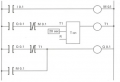# PLC diagram to logic equation and truth table

#### Matherios

Joined Feb 25, 2018
74
Hello I'm having a really hard time making the logic equation and the truth table of this Plc diagram.
So far i have went till this part of the equation.
• Q0.1=(Q0.1*(I0.1 * ^Q0.1))+I0.1=>
• Q0.1=(Q0.1*I0.1)+I0.1
• (^)=Inverse
I don't know if im correct with the equation but it's the first time i have the output of a circuit as an input aswell of the same equation.#### bertus

Joined Apr 5, 2008
21,856
Hello,

Bertus

Joined Jul 18, 2013
24,995
I am not that familiar with programming ladder logic in Boolean, most PLC's can be programmed in an alternative Step style language that resembles Boolean in a way, and an output function used in a logic function would have the same address as the output.
Max.

Joined Jul 18, 2013
24,995
Most likely treated exactly the same way as it is in the ladder format, IOW, although being shown as a condition inside the rung, the address is the same. and treated the same as an input would be.
Max.

#### Matherios

Joined Feb 25, 2018
74
What is IOW?
Also the Q0.1 switch (not the relay) depends on the condition of the Q0.1 (relay), for example when the relay is energized then the switch Q0.1 is closed.So how can i take Q0.1 switch as an input?

Joined Jul 18, 2013
24,995
IOW = In Other Words.
Normally you express it as just that, NOT/NOR Q0.1. etc.
Just as it is done in ladder.
Max.

#### Matherios

Joined Feb 25, 2018
74
Can you please expain it a bit more?

Joined Jul 18, 2013
24,995
• Q0.1=(Q0.1*(I0.1 * ^Q0.1))+I0.1=>
• Q0.1=(Q0.1*I0.1)+I0.1
• (^)=Inverse
I don't know if im correct with the equation but it's the first time i have the output of a circuit as an input aswell of the same equation.
On review it looks like you have it right, What is your reference material you are using?
Any PLC make in particular?
Max.

#### Matherios

Joined Feb 25, 2018
74
Our teacher just gave us a random plc diagram,so i don't know in particular the specific plc.

Joined Jul 18, 2013
24,995
But did you get that form of ladder to Boolean conversion from any particular document?
Max.

#### Matherios

Joined Feb 25, 2018
74
No,i just got the random ladder diagram and converted just as i knew.